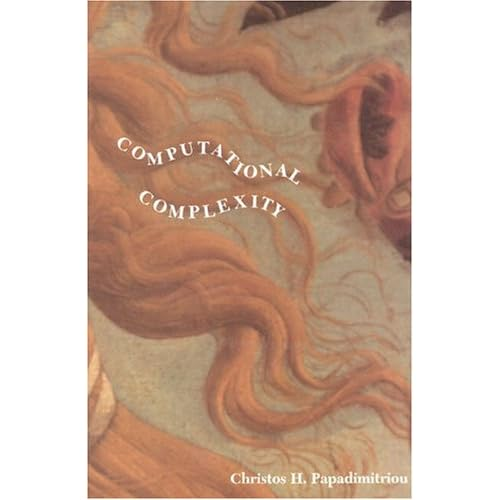Geeks With Blogs

I just re-read Complexity – A Guided Tour by Melanie Mitchell , protégé of Douglas Hofstadter ( author of “Gödel, Escher, Bach”)

http://www.amazon.com/Complexity-Guided-Tour-Melanie-Mitchell/dp/0199798109/ref=sr_1_1?ie=UTF8&qid=1339744329&sr=8-1here are some notes and links:

Evolved from Cybernetics, General Systems Theory, Synergetics

some interesting transdisciplinary fields to investigate:

There are limits to deterministic systems & to computation.

Chaos Theory definitely applies to training an ANN (artificial neural network) – different weights will emerge depending upon the random selection of the training set.

In recursive Non-Linear systems http://en.wikipedia.org/wiki/Nonlinear_system – output is not directly inferable from input. E.g. a Logistic map: Xt+1 = R Xt(1-Xt)

Different types of bifurcations, attractor states and oscillations may occur – e.g. a Lorenz Attractor http://en.wikipedia.org/wiki/Lorenz_system

Feigenbaum Constants http://en.wikipedia.org/wiki/Feigenbaum_constants express ratios in a bifurcation diagram for a non-linear map – the convergent limit of R (the rate of period-doubling bifurcations) is 4.6692016

Maxwell’s Demon - http://en.wikipedia.org/wiki/Maxwell%27s_demon - the Second Law of Thermodynamics has only a statistical certainty – the universe (and thus information) tends towards entropy. While any computation can theoretically be done without expending energy, with finite memory, the act of erasing memory is permanent and increases entropy. Life & thought is a counter-example to the universe’s tendency towards entropy.

Leo Szilard and later Claude Shannon came up with the Information Theory of Entropy - http://en.wikipedia.org/wiki/Entropy_(information_theory) whereby Shannon entropy quantifies the expected value of a message’s information in bits in order to determine channel capacity and leverage Coding Theory (compression analysis).

Ludwig Boltzmann came up with Statistical Mechanics - http://en.wikipedia.org/wiki/Statistical_mechanics – whereby our Newtonian perception of continuous reality is a probabilistic and statistical aggregate of many discrete quantum microstates. This is relevant for Quantum Information Theory http://en.wikipedia.org/wiki/Quantum_information and the Physics of Information - http://en.wikipedia.org/wiki/Physical_information.

Hilbert’s Problems http://en.wikipedia.org/wiki/Hilbert's_problems pondered whether mathematics is complete, consistent, and decidable (the Decision Problemhttp://en.wikipedia.org/wiki/Entscheidungsproblem – is there always an algorithm that can determine whether a statement is true).  Godel’s Incompleteness Theorems http://en.wikipedia.org/wiki/G%C3%B6del's_incompleteness_theorems  proved that mathematics cannot be both complete and consistent (e.g. “This statement is not provable”). Turing through the use of Turing Machines (http://en.wikipedia.org/wiki/Turing_machine symbol processors that can prove mathematical statements) and Universal Turing Machines (http://en.wikipedia.org/wiki/Universal_Turing_machine Turing Machines that can emulate other any Turing Machine via accepting programs as well as data as input symbols) that computation is limited by demonstrating the Halting Problem http://en.wikipedia.org/wiki/Halting_problem (is is not possible to know when a program will complete – you cannot build an infinite loop detector).

You may be used to thinking of 1 / 2 / 3 dimensional systems, but Fractal http://en.wikipedia.org/wiki/Fractal systems are defined by self-similarity & have non-integer Hausdorff Dimensions !!!  http://en.wikipedia.org/wiki/List_of_fractals_by_Hausdorff_dimension – the fractal dimension quantifies the number of copies of a self similar object at each level of detail – eg Koch Snowflake - http://en.wikipedia.org/wiki/Koch_snowflake

Definitions of complexity: size, Shannon entropy, Algorithmic Information Content (http://en.wikipedia.org/wiki/Algorithmic_information_theory - size of shortest program that can generate a description of an object) Logical depth (amount of info processed), thermodynamic depth (resources required). Complexity is statistical and fractal.

John Von Neumann’s other machine was the Self-Reproducing Automaton http://en.wikipedia.org/wiki/Self-replicating_machine  . Cellular Automata http://en.wikipedia.org/wiki/Cellular_automaton are alternative form of Universal Turing machine to traditional Von Neumann machines where grid cells are locally synchronized with their neighbors according to a rule. Conway’s Game of Life http://en.wikipedia.org/wiki/Conway's_Game_of_Life demonstrates various emergent constructs such as “Glider Guns” and “Spaceships”. Cellular Automatons are not practical because logical ops require a large number of cells – wasteful & inefficient. There are no compilers or general program languages available for Cellular Automatons (as far as I am aware). Random Boolean Networks http://en.wikipedia.org/wiki/Boolean_network are extensions of cellular automata where nodes are connected at random (not to spatial neighbors) and each node has its own rule –> they demonstrate the emergence of complex  & self organized behavior.

Stephen Wolfram’s (creator of Mathematica, so give him the benefit of the doubt) New Kind of Science http://en.wikipedia.org/wiki/A_New_Kind_of_Science proposes the universe may be a discrete Finite State Automata http://en.wikipedia.org/wiki/Finite-state_machine whereby reality emerges from simple rules. I am 2/3 through this book. It is feasible that the universe is quantum discrete at the plank scale and that it computes itself – Digital Physics: http://en.wikipedia.org/wiki/Digital_physics – a simulated reality? Anyway, all behavior is supposedly derived from simple algorithmic rules & falls into 4 patterns: uniform , nested / cyclical, random (Rule 30 http://en.wikipedia.org/wiki/Rule_30) & mixed (Rule 110 - http://en.wikipedia.org/wiki/Rule_110 localized structures – it is this that is interesting). interaction between colliding propagating signal inputs is then information processing. Wolfram proposes the Principle of Computational Equivalence - http://mathworld.wolfram.com/PrincipleofComputationalEquivalence.html - all processes that are not obviously simple can be viewed as computations of equivalent sophistication.

Meaning in information may emerge from analogy & conceptual slippages – see the CopyCat program: http://cognitrn.psych.indiana.edu/rgoldsto/courses/concepts/copycat.pdf

Scale Free Networks http://en.wikipedia.org/wiki/Scale-free_network have a distribution governed by a Power Law (http://en.wikipedia.org/wiki/Power_law - much more common than Normal Distribution). They are characterized by hubs (resilience to random deletion of nodes), heterogeneity of degree values, self similarity, & small world structure. They grow via preferential attachment http://en.wikipedia.org/wiki/Preferential_attachment – tipping points triggered by positive feedback loops. 2 theories of cascading system failures in complex systems are Self-Organized Criticality http://en.wikipedia.org/wiki/Self-organized_criticality and Highly Optimized Tolerance http://en.wikipedia.org/wiki/Highly_optimized_tolerance.

Computational Mechanics http://en.wikipedia.org/wiki/Computational_mechanics – use of computational methods to study phenomena governed by the principles of mechanics.

This book is a great intuition pump, but does not cover the more mathematical subject of Computational Complexity Theoryhttp://en.wikipedia.org/wiki/Computational_complexity_theory I am currently reading this book on this subject: http://www.amazon.com/Computational-Complexity-Christos-H-Papadimitriou/dp/0201530821/ref=pd_sim_b_1stay tuned for that review!